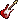» » »

# Chapman Tracy - If Not Now Tabs

Artists:  A B C D E F G H I J K L M N O P Q R S T U V W X Y Z #

## If Not Now Tabs

Chapman Tracy

```Tabs too difficult? Try these video lessons and learn fast
```
```                {title:If Not Now}
{st:by Tracy Chapman}
{c:capo barred at the second fret}

{c: Riff:}
Gmaj7                                    D
F=====0===0==0===0==|0==0====0====2=====|0====0==0======0==|0==0====0===0====
C#=T==1===1==1===1==|0==1====1======3===|1====1==1======1==|0==1====1=====1==
A==A==2===2==2===2==|2==2====2========2=|0====0==0======0==|0==0====0=======0
E==B==3===3==3===3==|3==3====3==========|2====2==2======2==|2==2====2========
B===================|===================|3====3==3======3==|3==3====3========
F===================|===================|==================|=================
If not now then when
If not today then
[G]A love declared for days to [Em]come
(133211)Is as good as none

You can wait 'til morning comes
You can wait for the new day
You can wait and lose this heart
You can wait and soon be sorry

Now love's the only thing that's free
We must take it where it's found
Pretty soon it may be costly

If not now what then
We all must live out lives
Always feeling
Always thinking
The moment has arrived
```
`Submit corrections`

```Hard to play? Try these video lessons and learn fast```
↑ Back to top | Tablatures and chords for acoustic guitar and electric guitar, ukulele, drums are parodies/interpretations of the original songs. You may use it for private study, scholarship, research or language learning purposes only